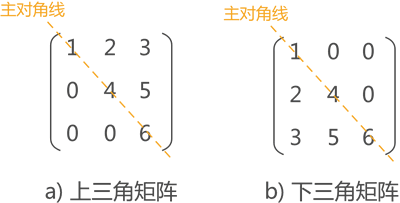【算法工程师的数学基础】系列将会从线性代数、微积分、数值优化、概率论、信息论五个方面进行介绍，感兴趣的欢迎关注【搜索与推荐Wiki】公众号，获得最新文章。

《算法工程师的数学基础》已更新：

### 矩阵操作

• 结合律：$(AB)C = A(BC)$
• 分配律：$(A+B)C = AC+BC, C(A+B)=CA + CB$

Hadamard积 $A$和$B$的Hadamard积，也称为逐点乘积，为$A$和$B$中对应的元素相乘。

### 矩阵类型$n*n$的对角矩阵$A=diag(a)$和$n$维向量$b$的乘积为一个$n$维向量

Gram矩阵 向量空间中一组向量$v_1, v_2, …, v_n$的Gram矩阵，$G$是内积的对称矩阵，其元素$G_{ij}$为$v_i^T v_j$

### 矩阵分解

【技术服务】，详情点击查看： https://mp.weixin.qq.com/s/PtX9ukKRBmazAWARprGIAg